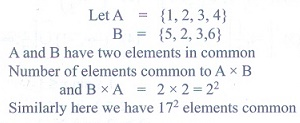# Chapter 1: Sets, Relations and Functions - Online Test

Q1. If A = {(x, y) : y = ex, xR} and B = {(x, y) : y = e-x, x R} then n(A B) is
Explaination / Solution:Q2. If A = {(x, y) : y = sin x, x R} and B = {(x, y) : y = cos x, x R} then A B contains
Explaination / Solution:
No Explaination.

Q3. The relation R defined on a set A = {0,-1, 1, 2} by xRy if |x2 + y2| ≤  2, then which one of the following is true?
Explaination / Solution: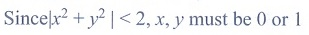Q4. If {(x) = |x 2| + |x + 2|, x R, then
Explaination / Solution:Q5.

Let R be the set of all real numbers. Consider the following subsets of the plane R x  R:

S = {(x, y) : y = x + 1 and 0 < x < 2} and T = {(x, y) : x - y is an integer }

Then which of the following is true?
Explaination / Solution: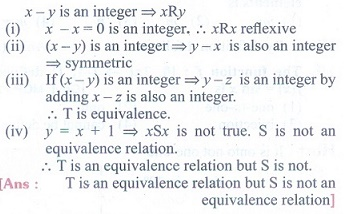Q6. Let A and B be subsets of the universal set N, the set of natural numbers. Then A’ [(AB)B’] is
Explaination / Solution: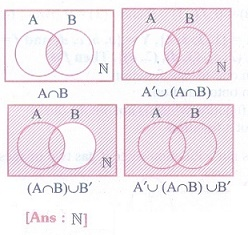Q7. The number of students who take both the subjects Mathematics and Chemistry is 70. This represents 10% of the enrollment in Mathematics and 14% of the enrollment in Chemistry. The number of students take at least one of these two subjects, is
Explaination / Solution: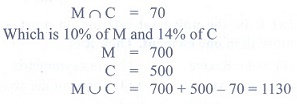Q8. If n((A x B) (A x C)) = 8 and n(B C) = 2, then n(A) is
Explaination / Solution: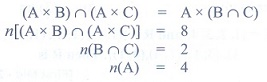Q9. If n(A) = 2 and n(B C) = 3, then n[(A x B) (A xC)] is
Explaination / Solution:Q10.

If two sets A and B have 17 elements in common, then the number of elements common to the set A x B and B x A is

Explaination / Solution: